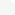# Categories# E-Books →Distribution Theory and Transform Analysis: An Introduction to Generalized Functions, with ApplicationsA.H. Zemanian, "Distribution Theory and Transform Analysis: An Introduction to Generalized Functions, with Applications"
2010 | ISBN-10: 0486654796 | 400 pages | EPUB | 24 MB

Distribution theory, a relatively recent mathematical approach to classical Fourier analysis, not only opened up new areas of research but also helped promote the development of such mathematical disciplines as ordinary and partial differential equations, operational calculus, transformation theory, and functional analysis. This text was one of the first to give a clear explanation of distribution theory; it combines the theory effectively with extensive practical applications to science and engineering problems.
Based on a graduate course given at the State University of New York at Stony Brook, this book has two objectives: to provide a comparatively elementary introduction to distribution theory and to describe the generalized Fourier and Laplace transformations and their applications to integrodifferential equations, difference equations, and passive systems.
After an introductory chapter defining distributions and the operations that apply to them, Chapter 2 considers the calculus of distributions, especially limits, differentiation, integrations, and the interchange of limiting processes. Some deeper properties of distributions, such as their local character as derivatives of continuous functions, are given in Chapter 3. Chapter 4 introduces the distributions of slow growth, which arise naturally in the generalization of the Fourier transformation. Chapters 5 and 6 cover the convolution process and its use in representing differential and difference equations. The distributional Fourier and Laplace transformations are developed in Chapters 7 and 8, and the latter transformation is applied in Chapter 9 to obtain an operational calculus for the solution of differential and difference equations of the initial-condition type. Some of the previous theory is applied in Chapter 10 to a discussion of the fundamental properties of certain physical systems, while Chapter 11 ends the book with a consideration of periodic distributions.
Suitable for a graduate course for engineering and science students or for a senior-level undergraduate course for mathematics majors, this book presumes a knowledge of advanced calculus and the standard theorems on the interchange of limit processes. A broad spectrum of problems has been included to satisfy the diverse needs of various types of students.

# Search

«    July 2019    »
MonTueWedThuFriSatSun
1234567
891011121314
15161718192021
22232425262728
293031

» ReleaseLog
» 0dayHome